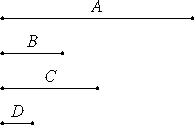# Proposition 38

If a number has any part whatever, then it is measured by a number called by the same name as the part.

Let the number A have any part whatever, B, and let C be a number called by the same name as the part B.I say that C measures A.

Since B is a part of A called by the same name as C, and the unit D is also a part of C called by the same name as it, therefore the part B of A is the same part of the unit D of the number C. Therefore the unit D measures the number C the same number of times that B measures A.

VII.15

Therefore, alternately, the unit D measures the number B the same number of times that C measures A. Therefore C measures A.

Therefore, if a number has any part whatever, then it is measured by a number called by the same name as the part.

Q.E.D.

## Guide

This proposition says that if a has a one-cth part of a, then c divides a. For example, 12 has a one-third part, 3 divides 12. This is a converse of the last proposition.

#### Use of this proposition.

This proposition is used in the proof of the next proposition.